## Cache Mapping-

Before you go through this article, make sure that you have gone through the previous article on Cache Mapping.

We have discussed-

• Cache mapping is a technique by which the contents of main memory are brought into the cache.
• Cache mapping is performed using following three different techniques-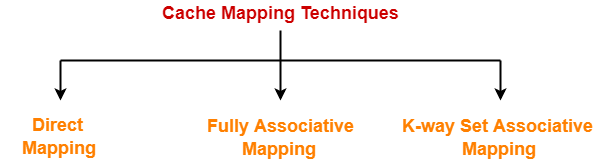In this article, we will discuss practice problems based on cache mapping techniques.

## Problem-01:

The main memory of a computer has 2 cm blocks while the cache has 2c blocks. If the cache uses the set associative mapping scheme with 2 blocks per set, then block k of the main memory maps to the set-

1. (k mod m) of the cache
2. (k mod c) of the cache
3. (k mod 2 c) of the cache
4. (k mod 2 cm) of the cache

## Solution-

Given-

• Number of blocks in main memory = 2 cm
• Number of blocks in cache = 2 c
• Number of blocks in one set of cache = 2

### Number of Sets in Cache-

Number of sets in cache

= Number of blocks in cache / Number of blocks in one set

= 2 c / 2

= c

### Required Mapping-

In set associative mapping,

• Block ‘j’ of main memory maps to set number (j modulo number of sets in cache) of the cache.
• So, block ‘k’ of main memory maps to set number (k mod c) of the cache.
• Thus, option (B) is correct.

Also Read- Practice Problems On Direct Mapping

## Problem-02:

In a k-way set associative cache, the cache is divided into v sets, each of which consists of k lines. The lines of a set placed in sequence one after another. The lines in set s are sequenced before the lines in set (s+1). The main memory blocks are numbered 0 on wards. The main memory block numbered ‘j’ must be mapped to any one of the cache lines from-

1. (j mod v) x k to (j mod v) x k + (k – 1)
2. (j mod v) to (j mod v) + (k – 1)
3. (j mod k) to (j mod k) + (v – 1)
4. (j mod k) x v to (j mod k) x v + (v – 1)

## Solution-

Let-

• 2-way set associative mapping is used, then k = 2
• Number of sets in cache = 4, then v = 4
• Block number ‘3’ has to be mapped, then j = 3

The given options on substituting these values reduce to-

1. (3 mod 4) x 2 to (3 mod 4) x 2 + (2 – 1) = 6 to 7
2. (3 mod 4) to (3 mod 4) + (2 – 1) = 3 to 4
3. (3 mod 2) to (3 mod 2) + (4 – 1) = 1 to 4
4. (3 mod 2) x 4 to (3 mod 2) x 4 + (4 – 1) = 4 to 7

Now, we have been asked to what range of cache lines, block number 3 can be mapped.

According to the above data, the cache memory and main memory will look like-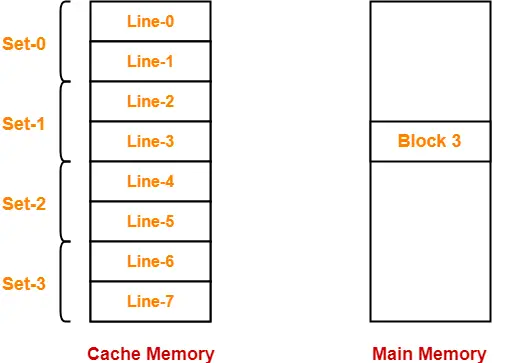In set associative mapping,

• Block ‘j’ of main memory maps to set number (j modulo number of sets in cache) of the cache.
• So, block 3 of main memory maps to set number (3 mod 4) = 3 of cache.
• Within set number 3, block 3 can be mapped to any of the cache lines.
• Thus, block 3 can be mapped to cache lines ranging from 6 to 7.

Thus, Option (A) is correct.

## Problem-03:

A block-set associative cache memory consists of 128 blocks divided into four block sets . The main memory consists of 16,384 blocks and each block contains 256 eight bit words.

1. How many bits are required for addressing the main memory?
2. How many bits are needed to represent the TAG, SET and WORD fields?

## Solution-

Given-

• Number of blocks in cache memory = 128
• Number of blocks in each set of cache = 4
• Main memory size = 16384 blocks
• Block size = 256 bytes
• 1 word = 8 bits = 1 byte

### Main Memory Size-

We have-

Size of main memory

= 16384 blocks

= 16384 x 256 bytes

= 222 bytes

Thus, Number of bits required to address main memory = 22 bits

### Number of Bits in Block Offset-

We have-

Block size

= 256 bytes

= 28 bytes

Thus, Number of bits in block offset or word = 8 bits

### Number of Bits in Set Number-

Number of sets in cache

= Number of lines in cache / Set size

= 128 blocks / 4 blocks

= 32 sets

= 25 sets

Thus, Number of bits in set number = 5 bits

### Number of Bits in Tag Number-

Number of bits in tag

= Number of bits in physical address – (Number of bits in set number + Number of bits in word)

= 22 bits – (5 bits + 8 bits)

= 22 bits – 13 bits

= 9 bits

Thus, Number of bits in tag = 9 bits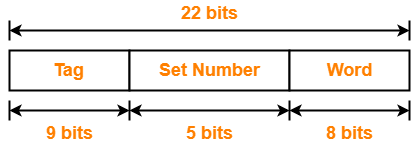## Problem-04:

A computer has a 256 KB, 4-way set associative, write back data cache with block size of 32 bytes. The processor sends 32 bit addresses to the cache controller. Each cache tag directory entry contains in addition to address tag, 2 valid bits, 1 modified bit and 1 replacement bit.

### Part-01:

The number of bits in the tag field of an address is-

1. 11
2. 14
3. 16
4. 27

### Part-02:

The size of the cache tag directory is-

1. 160 Kbits
2. 136 Kbits
3. 40 Kbits
4. 32 Kbits

## Solution-

Given-

• Cache memory size = 256 KB
• Set size = 4 blocks
• Block size = 32 bytes
• Number of bits in physical address = 32 bits

### Number of Bits in Block Offset-

We have-

Block size

= 32 bytes

= 25 bytes

Thus, Number of bits in block offset = 5 bits

### Number of Lines in Cache-

Number of lines in cache

= Cache size / Line size

= 256 KB / 32 bytes

= 218 bytes / 25 bytes

= 213 lines

Thus, Number of lines in cache = 213 lines

### Number of Sets in Cache-

Number of sets in cache

= Number of lines in cache / Set size

= 213 lines / 22 lines

= 211 sets

Thus, Number of bits in set number = 11 bits

### Number of Bits in Tag-

Number of bits in tag

= Number of bits in physical address – (Number of bits in set number + Number of bits in block offset)

= 32 bits – (11 bits + 5 bits)

= 32 bits – 16 bits

= 16 bits

Thus, Number of bits in tag = 16 bits

### Tag Directory Size-

Size of tag directory

= Number of lines in cache x Size of tag

= 213 x (16 bits + 2 valid bits + 1 modified bit + 1 replacement bit)

= 213 x 20 bits

= 163840 bits

= 20 KB or 160 Kbits

Thus,

• For part-01, Option (C) is correct.
• For part-02, Option (A) is correct.

## Problem-05:

A 4-way set associative cache memory unit with a capacity of 16 KB is built using a block size of 8 words. The word length is 32 bits. The size of the physical address space is 4 GB. The number of bits for the TAG field is _____.

## Solution-

Given-

• Set size = 4 lines
• Cache memory size = 16 KB
• Block size = 8 words
• 1 word = 32 bits = 4 bytes
• Main memory size = 4 GB

### Number of Bits in Physical Address-

We have,

Main memory size

= 4 GB

= 232 bytes

Thus, Number of bits in physical address = 32 bits

### Number of Bits in Block Offset-

We have,

Block size

= 8 words

= 8 x 4 bytes

= 32 bytes

= 25 bytes

Thus, Number of bits in block offset = 5 bits

### Number of Lines in Cache-

Number of lines in cache

= Cache size / Line size

= 16 KB / 32 bytes

= 214 bytes / 25 bytes

= 29 lines

= 512 lines

Thus, Number of lines in cache = 512 lines

### Number of Sets in Cache-

Number of sets in cache

= Number of lines in cache / Set size

= 512 lines / 4 lines

= 29 lines / 22 lines

= 27 sets

Thus, Number of bits in set number = 7 bits

### Number of Bits in Tag-

Number of bits in tag

= Number of bits in physical address – (Number of bits in set number + Number of bits in block offset)

= 32 bits – (7 bits + 5 bits)

= 32 bits – 12 bits

= 20 bits

Thus, number of bits in tag = 20 bits

## Problem-06:

If the associativity of a processor cache is doubled while keeping the capacity and block size unchanged, which one of the following is guaranteed to be NOT affected?

1. Width of tag comparator
2. Width of set index decoder
3. Width of way selection multiplexer
4. Width of processor to main memory data bus

## Solution-

Since block size is unchanged, so number of bits in block offset will remain unchanged.

### Effect On Width Of Tag Comparator-

• Associativity of cache is doubled means number of lines in one set is doubled.
• Since number of lines in one set is doubled, therefore number of sets reduces to half.
• Since number of sets reduces to half, so number of bits in set number decrements by 1.
• Since number of bits in set number decrements by 1, so number of bits in tag increments by 1.
• Since number of bits in tag increases, therefore width of tag comparator also increases.

### Effect On Width of Set Index Decoder-

• Associativity of cache is doubled means number of lines in one set is doubled.
• Since number of lines in one set is doubled, therefore number of sets reduces to half.
• Since number of sets reduces to half, so number of bits in set number decrements by 1.
• Since number of bits in set number decreases, therefore width of set index decoder also decreases.

### Effect On Width Of Way Selection Multiplexer-

• Associativity of cache (k) is doubled means number of lines in one set is doubled.
• New associativity of cache is 2k.
• To handle new associativity, size of multiplexers must be 2k x 1.
• Therefore, width of way selection multiplexer increases.

### Effect On Width Of Processor To Main Memory Data Bus-

• Processor to main memory data bus has nothing to do with cache associativity.
• It depends on the number of bits in block offset which is unchanged here.
• So, width of processor to main memory data bus is not affected and remains unchanged.

Thus, Option (D) is correct.

## Problem-07:

Consider a direct mapped cache with 8 cache blocks (0-7). If the memory block requests are in the order-

3, 5, 2, 8, 0, 6, 3, 9, 16, 20, 17, 25, 18, 30, 24, 2, 63, 5, 82, 17, 24

Which of the following memory blocks will not be in the cache at the end of the sequence?

1. 3
2. 18
3. 20
4. 30

Also, calculate the hit ratio and miss ratio.

## Solution-

We have,

• There are 8 blocks in cache memory numbered from 0 to 7.
• In direct mapping, a particular block of main memory is mapped to a particular line of cache memory.
• The line number is given by-

Cache line number = Block address modulo Number of lines in cache

For the given sequence-

• Requests for memory blocks are generated one by one.
• The line number of the block is calculated using the above relation.
• Then, the block is placed in that particular line.
• If already there exists another block in that line, then it is replaced.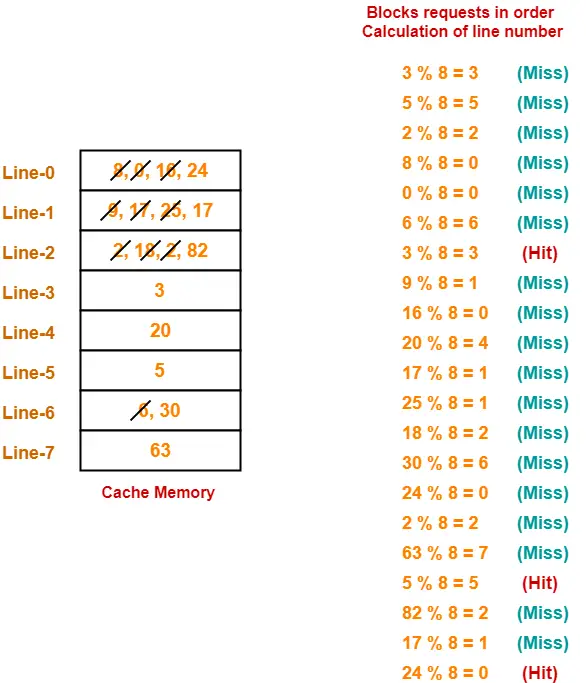Thus,

• Out of given options, only block-18 is not present in the main memory.
• Option-(B) is correct.
• Hit ratio = 3 / 20
• Miss ratio = 17 / 20

## Problem-08:

Consider a fully associative cache with 8 cache blocks (0-7). The memory block requests are in the order-

4, 3, 25, 8, 19, 6, 25, 8, 16, 35, 45, 22, 8, 3, 16, 25, 7

If LRU replacement policy is used, which cache block will have memory block 7?

Also, calculate the hit ratio and miss ratio.

## Solution-

We have,

• There are 8 blocks in cache memory numbered from 0 to 7.
• In fully associative mapping, any block of main memory can be mapped to any line of the cache that is freely available.
• If all the cache lines are already occupied, then a block is replaced in accordance with the replacement policy.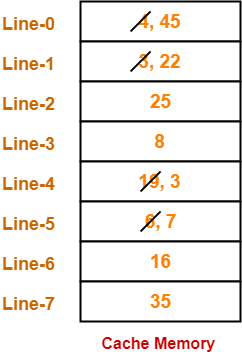Thus,

• Line-5 contains the block-7.
• Hit ratio = 5 / 17
• Miss ratio = 12 / 17

## Problem-09:

Consider a 4-way set associative mapping with 16 cache blocks. The memory block requests are in the order-

0, 255, 1, 4, 3, 8, 133, 159, 216, 129, 63, 8, 48, 32, 73, 92, 155

If LRU replacement policy is used, which cache block will not be present in the cache?

1. 3
2. 8
3. 129
4. 216

Also, calculate the hit ratio and miss ratio.

## Solution-

We have,

• There are 16 blocks in cache memory numbered from 0 to 15.
• Each set contains 4 cache lines.
• In set associative mapping, a particular block of main memory is mapped to a particular set of cache memory.
• The set number is given by-

Cache line number = Block address modulo Number of sets in cache

For the given sequence-

• Requests for memory blocks are generated one by one.
• The set number of the block is calculated using the above relation.
• Within that set, the block is placed in any freely available cache line.
• If all the blocks are already occupied, then one of the block is replaced in accordance with the employed replacement policy.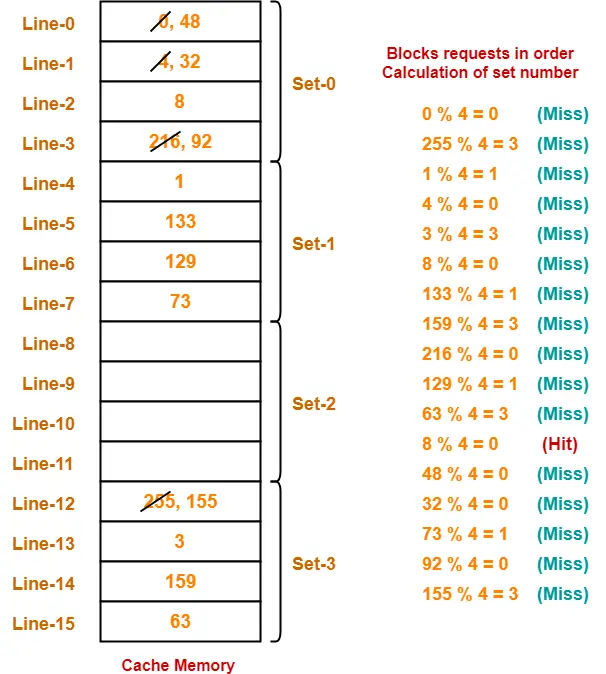Thus,

• Out of given options, only block-216 is not present in the main memory.
• Option-(D) is correct.
• Hit ratio = 1 / 17
• Miss ratio = 16 / 17

## Problem-10:

Consider a small 2-way set associative mapping with a total of 4 blocks. LRU replacement policy is used for choosing the block to be replaced. The number of cache misses for the following sequence of block addresses 8, 12, 0, 12, 8 is ____.

## Solution-

• Practice yourself.
• Total number of cache misses = 4

## Problem-11:

Consider the cache has 4 blocks. For the memory references-

5, 12, 13, 17, 4, 12, 13, 17, 2, 13, 19, 13, 43, 61, 19

What is the hit ratio for the following cache replacement algorithms-

1. FIFO
2. LRU
3. Direct mapping
4. 2-way set associative mapping using LRU

## Solution-

• Practice yourself.
• Using FIFO as cache replacement algorithm, hit ratio = 5/15 = 1/3.
• Using LRU as cache replacement algorithm, hit ratio = 6/15 = 2/5.
• Using direct mapping as cache replacement algorithm, hit ratio = 1/15.
• Using 2-way set associative mapping as cache replacement algorithm, hit ratio = 5/15 = 1/3

## Problem-12:

A hierarchical memory system has the following specification, 20 MB main storage with access time of 300 ns, 256 bytes cache with access time of 50 ns, word size 4 bytes, page size 8 words. What will be the hit ratio if the page address trace of a program has the pattern 0, 1, 2, 3, 0, 1, 2, 4 following LRU page replacement technique?

## Solution-

Given-

• Main memory size = 20 MB
• Main memory access time = 300 ns
• Cache memory size = 256 bytes
• Cache memory access time = 50 ns
• Word size = 4 bytes
• Page size = 8 words

We have,

Line size

= 8 words

= 8 x 4 bytes

= 32 bytes

### Number of Lines in Cache-

Number of lines in cache

= Cache size / Line size

= 256 bytes / 32 bytes

= 8 lines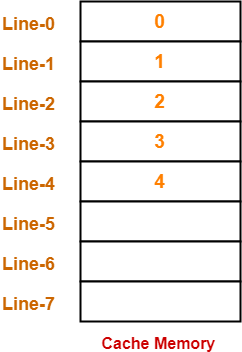Thus,

• Number of hits = 3
• Hit ratio = 3 / 8

## Problem-13:

Consider an array A and each element occupies 4 words. A 32 word cache is used and divided into 8 word blocks. What is the hit ratio for the following code-

for (i=0 ; i < 100 ; i++)

A[i] = A[i] + 10;

## Solution-

Number of lines in cache

= Cache size / Line size

= 32 words / 8 words

= 4 lines

Since each element of the array occupies 4 words, so-

Number of elements that can be placed in one line = 2

Now, let us analyze the statement-

A[i] = A[i] + 10;

For each i,

• Firstly, the value of A[i] is read.
• Secondly, 10 is added to the A[i].
• Thirdly, the updated value of A[i] is written back.

Thus,

• For each i, A[i] is accessed two times.
• Two memory accesses are required-one for read operation and other for write operation.

Assume the cache is all empty initially.

In the first loop iteration,

• First, value of A has to be read.
• Since cache is empty, so A is brought in the cache.
• Along with A, element A also enters the cache since each block can hold 2 elements of the array.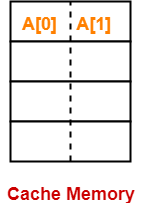• Thus, For A, a miss occurred for the read operation.
• Now, 10 is added to the value of A.
• Now, the updated value has to be written back to A.
• Again cache memory is accessed to get A for writing its updated value.
• This time, for A, a hit occurs for the write operation.

In the second loop iteration,

• First, value of A has to be read.
• A is already present in the cache.
• Thus, For A. a hit occurs for the read operation.
• Now, 10 is added to the value of A.
• Now, the updated value has to be written back to A.
• Again cache memory is accessed to get A for writing its updated value.
• Again, for A, a hit occurs for the write operation.

In the similar manner, for every next two consecutive elements-

• There will be a miss for the read operation for the first element.
• There will be a hit for the write operation for the first element.
• There will be a hit for both read and write operations for the second element.

Likewise, for 100 elements, we will have 50 such pairs in cache and in every pair, there will be one miss and three hits.

Thus,

• Total number of hits = 50 x 3 = 150
• Total number of misses = 50 x 1 = 50
• Total number of references = 200 (100 for read and 100 for write)

Thus,

• Hit ratio = 150 / 200 = 3 / 4 = 0.75
• Miss ratio = 50 / 200 = 1 / 4 = 0.25

## Problem-14:

Consider an array has 100 elements and each element occupies 4 words. A 32 bit word cache is used and divided into a block of 8 words.

What is the hit ratio for the following statement-

for (i=0 ; i < 10 ; i++)

for (j=0 ; j < 10 ; j++)

A[i][j] = A[i][j] + 10;

if-

1. Row major order is used
2. Column major order is used

## Solution-

According to question, cache is divided as-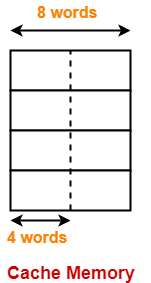In each cache line, two elements of the array can reside.

### Case-01: When Row Major Order is Used-

In row major order, elements of the array are stored row wise in the memory as-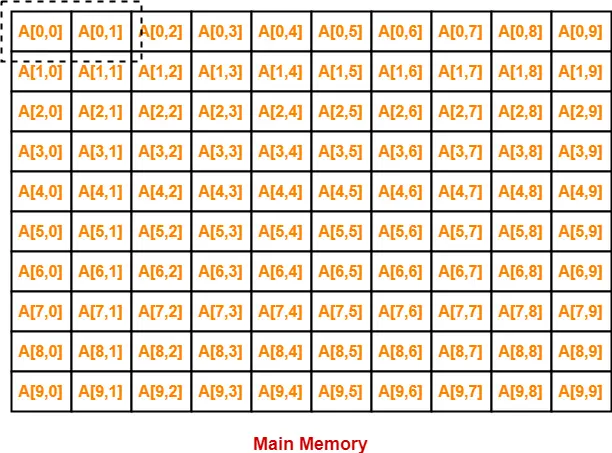• In the first iteration, when A will be brought in the cache, A will also be brought.
• Then, things goes as it went in the previous question.
• There will be a miss for A[0,0] read operation and hit for A[0,0] write operation.
• For A[0,1], there will be a hit for both read and write operations.
• Similar will be the story with every next two consecutive elements.

Thus,

• Total number of hits = 50 x 3 = 150
• Total number of misses = 50 x 1 = 50
• Total number of references = 200 (100 for read and 100 for write)

Thus,

• Hit ratio = 150 / 200 = 3 / 4 = 0.75
• Miss ratio = 50 / 200 = 1 / 4 = 0.25

### Case-02: When Column Major Order is Used-

In column major order, elements of the array are stored column wise in the memory as-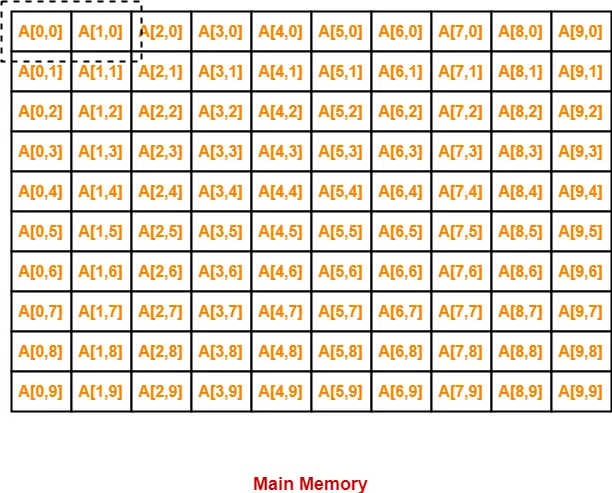• In the first iteration, when A[0,0] will be brought in the cache, A will also be brought.
• This time A will not be useful in iteration-2.
• A will be needed after surpassing 10 element.
• But by that time, this block would have got replaced since there are only 4 lines in the cache.
• For A to be useful, there has to be at least 10 lines in the cache.

Thus,

Under given scenario, for each element of the array-

• There will be a miss for the read operation.
• There will be a hit for the write operation.

Thus,

• Total number of hits = 100 x 1 = 100
• Total number of misses = 100 x 1 = 100
• Total number of references = 200 (100 for read and 100 for write)

Thus,

• Hit ratio = 100 / 200 = 1 / 2 = 0.50
• Miss ratio = 100 / 200 = 1 / 2 = 0.50

To increase the hit rate, following measures can be taken-

• Row major order can be used (Hit rate = 75%)
• The statement A[i][j] = A[i][j] + 10 can be replaced with A[j,i] = A[j][i] + 10 (Hit rate = 75%)

## Problem-15:

An access sequence of cache block addresses is of length N and contains n unique block addresses. The number of unique block addresses between two consecutive accesses to the same block address is bounded above by k. What is the miss ratio if the access sequence is passed through a cache of associativity A>=k exercising LRU replacement policy?

1. n/N
2. 1/N
3. 1/A
4. k/n

## Solution-

Required miss ratio = n/N.

Thus, Option (A) is correct.

Next Article- Cache Line | Effects of Changing Cache Line Size

Get more notes and other study material of Computer Organization and Architecture.

Watch video lectures by visiting our YouTube channel LearnVidFun.

## Direct Mapping-

Before you go through this article, make sure that you have gone through the previous article on Direct Mapping.

In direct mapping,

• A particular block of main memory can be mapped to one particular cache line only.
• Block ‘j’ of main memory will map to line number (j mod number of cache lines) of the cache.
• There is no need of any replacement algorithm.

In this article, we will discuss practice problems based on direct mapping.

## Problem-01:

Consider a direct mapped cache of size 16 KB with block size 256 bytes. The size of main memory is 128 KB. Find-

1. Number of bits in tag
2. Tag directory size

## Solution-

Given-

• Cache memory size = 16 KB
• Block size = Frame size = Line size = 256 bytes
• Main memory size = 128 KB

We consider that the memory is byte addressable.

### Number of Bits in Physical Address-

We have,

Size of main memory

= 128 KB

= 217 bytes

Thus, Number of bits in physical address = 17 bits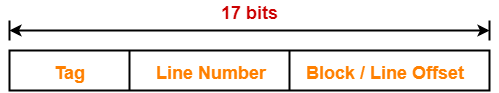### Number of Bits in Block Offset-

We have,

Block size

= 256 bytes

= 28 bytes

Thus, Number of bits in block offset = 8 bits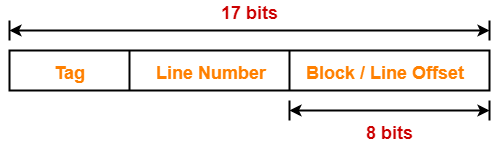### Number of Bits in Line Number-

Total number of lines in cache

= Cache size / Line size

= 16 KB / 256 bytes

= 214 bytes / 28 bytes

= 26 lines

Thus, Number of bits in line number = 6 bits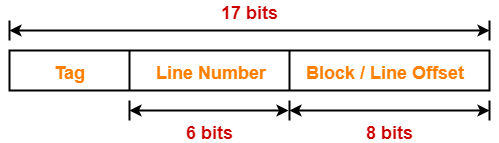### Number of Bits in Tag-

Number of bits in tag

= Number of bits in physical address – (Number of bits in line number + Number of bits in block offset)

= 17 bits – (6 bits + 8 bits)

= 17 bits – 14 bits

= 3 bits

Thus, Number of bits in tag = 3 bits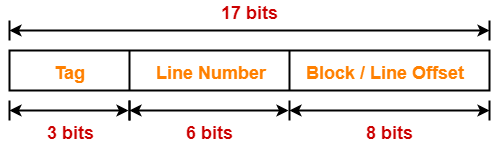### Tag Directory Size-

Tag directory size

= Number of tags x Tag size

= Number of lines in cache x Number of bits in tag

= 26 x 3 bits

= 192 bits

= 24 bytes

Thus, size of tag directory = 24 bytes

## Problem-02:

Consider a direct mapped cache of size 512 KB with block size 1 KB. There are 7 bits in the tag. Find-

1. Size of main memory
2. Tag directory size

## Solution-

Given-

• Cache memory size = 512 KB
• Block size = Frame size = Line size = 1 KB
• Number of bits in tag = 7 bits

We consider that the memory is byte addressable.

### Number of Bits in Block Offset-

We have,

Block size

= 1 KB

= 210 bytes

Thus, Number of bits in block offset = 10 bits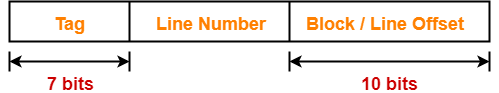### Number of Bits in Line Number-

Total number of lines in cache

= Cache size / Line size

= 512 KB / 1 KB

= 29 lines

Thus, Number of bits in line number = 9 bits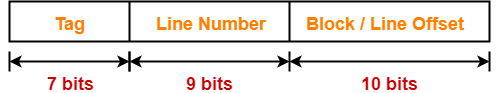### Number of Bits in Physical Address-

Number of bits in physical address

= Number of bits in tag + Number of bits in line number + Number of bits in block offset

= 7 bits + 9 bits + 10 bits

= 26 bits

Thus, Number of bits in physical address = 26 bits

### Size of Main Memory-

We have,

Number of bits in physical address = 26 bits

Thus, Size of main memory

= 226 bytes

= 64 MB

### Tag Directory Size-

Tag directory size

= Number of tags x Tag size

= Number of lines in cache x Number of bits in tag

= 29 x 7 bits

= 3584 bits

= 448 bytes

Thus, size of tag directory = 448 bytes

## Problem-03:

Consider a direct mapped cache with block size 4 KB. The size of main memory is 16 GB and there are 10 bits in the tag. Find-

1. Size of cache memory
2. Tag directory size

## Solution-

Given-

• Block size = Frame size = Line size = 4 KB
• Size of main memory = 16 GB
• Number of bits in tag = 10 bits

We consider that the memory is byte addressable.

### Number of Bits in Physical Address-

We have,

Size of main memory

= 16 GB

= 234 bytes

Thus, Number of bits in physical address = 34 bits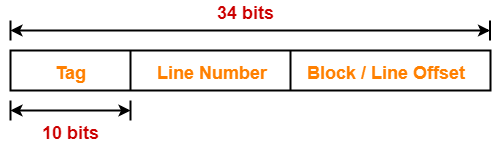### Number of Bits in Block Offset-

We have,

Block size

= 4 KB

= 212 bytes

Thus, Number of bits in block offset = 12 bits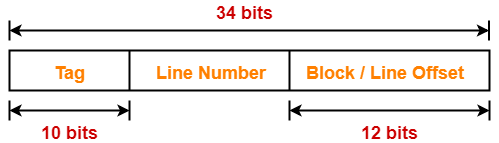### Number of Bits in Line Number-

Number of bits in line number

= Number of bits in physical address – (Number of bits in tag + Number of bits in block offset)

= 34 bits – (10 bits + 12 bits)

= 34 bits – 22 bits

= 12 bits

Thus, Number of bits in line number = 12 bits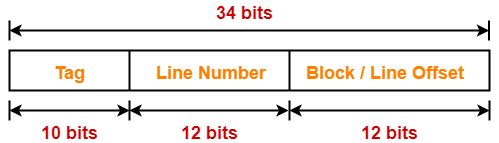### Number of Lines in Cache-

We have-

Number of bits in line number = 12 bits

Thus, Total number of lines in cache = 212 lines

### Size of Cache Memory-

Size of cache memory

= Total number of lines in cache x Line size

= 212 x 4 KB

= 214 KB

= 16 MB

Thus, Size of cache memory = 16 MB

### Tag Directory Size-

Tag directory size

= Number of tags x Tag size

= Number of lines in cache x Number of bits in tag

= 212 x 10 bits

= 40960 bits

= 5120 bytes

Thus, size of tag directory = 5120 bytes

## Problem-04:

Consider a direct mapped cache of size 32 KB with block size 32 bytes. The CPU generates 32 bit addresses. The number of bits needed for cache indexing and the number of tag bits are respectively-

1. 10, 17
2. 10, 22
3. 15, 17
4. 5, 17

## Solution-

Given-

• Cache memory size = 32 KB
• Block size = Frame size = Line size = 32 bytes
• Number of bits in physical address = 32 bits

### Number of Bits in Block Offset-

We have,

Block size

= 32 bytes

= 25 bytes

Thus, Number of bits in block offset = 5 bits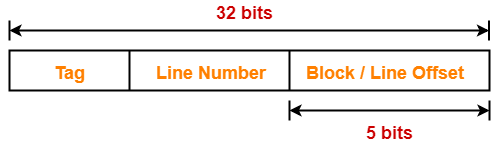### Number of Bits in Line Number-

Total number of lines in cache

= Cache size / Line size

= 32 KB / 32 bytes

= 210 lines

Thus, Number of bits in line number = 10 bits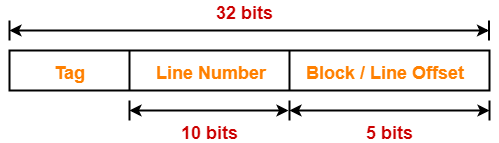### Number of Bits Required For Cache Indexing-

Number of bits required for cache indexing

= Number of bits in line number

= 10 bits

### Number Of Bits in Tag-

Number of bits in tag

= Number of bits in physical address – (Number of bits in line number + Number of bits in block offset)

= 32 bits – (10 bits + 5 bits)

= 32 bits – 15 bits

= 17 bits

Thus, Number of bits in tag = 17 bits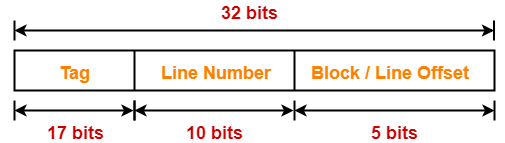Thus, Option (A) is correct.

## Problem-05:

Consider a machine with a byte addressable main memory of 232 bytes divided into blocks of size 32 bytes. Assume that a direct mapped cache having 512 cache lines is used with this machine. The size of the tag field in bits is ______.

## Solution-

Given-

• Main memory size = 232 bytes
• Block size = Frame size = Line size = 32 bytes
• Number of lines in cache = 512 lines

### Number of Bits in Physical Address-

We have,

Size of main memory

= 232 bytes

Thus, Number of bits in physical address = 32 bits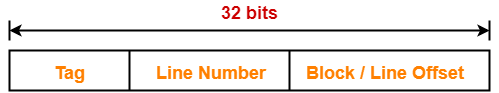### Number of Bits in Block Offset-

We have,

Block size

= 32 bytes

= 25 bytes

Thus, Number of bits in block offset = 5 bits### Number of Bits in Line Number-

Total number of lines in cache

= 512 lines

= 29 lines

Thus, Number of bits in line number = 9 bits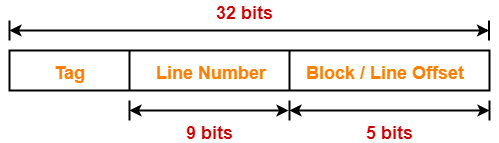### Number Of Bits in Tag-

Number of bits in tag

= Number of bits in physical address – (Number of bits in line number + Number of bits in block offset)

= 32 bits – (9 bits + 5 bits)

= 32 bits – 14 bits

= 18 bits

Thus, Number of bits in tag = 18 bits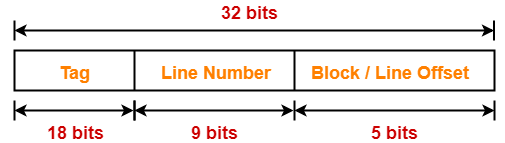## Problem-06:

An 8 KB direct-mapped write back cache is organized as multiple blocks, each of size 32 bytes. The processor generates 32 bit addresses. The cache controller maintains the tag information for each cache block comprising of the following-

• 1 valid bit
• 1 modified bit
• As many bits as the minimum needed to identify the memory block mapped in the cache

What is the total size of memory needed at the cache controller to store meta data (tags) for the cache?

1. 4864 bits
2. 6144 bits
3. 6656 bits
4. 5376 bits

## Solution-

Given-

• Cache memory size = 8 KB
• Block size = Frame size = Line size = 32 bytes
• Number of bits in physical address = 32 bits

### Number of Bits in Block Offset-

We have,

Block size

= 32 bytes

= 25 bytes

Thus, Number of bits in block offset = 5 bits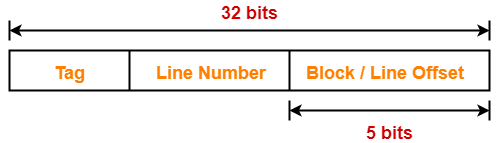### Number of Bits in Line Number-

Total number of lines in cache

= Cache memory size / Line size

= 8 KB / 32 bytes

= 213 bytes / 25 bytes

= 28 lines

Thus, Number of bits in line number = 8 bits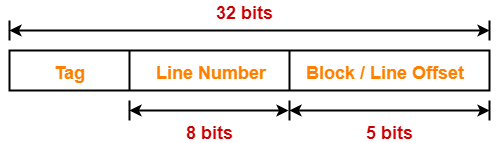### Number Of Bits in Tag-

Number of bits in tag

= Number of bits in physical address – (Number of bits in line number + Number of bits in block offset)

= 32 bits – (8 bits + 5 bits)

= 32 bits – 13 bits

= 19 bits

Thus, Number of bits in tag = 19 bits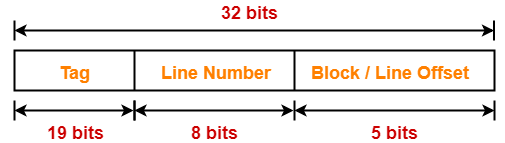### Memory Size Needed At Cache Controller-

Size of memory needed at cache controller

= Number of lines in cache x (1 valid bit + 1 modified bit + 19 bits to identify block)

= 28 x 21 bits

= 5376 bits

To watch video solutions and practice more problems,

Watch this Video Lecture

Next Article- Practice Problems On Fully Associative Mapping

Get more notes and other study material of Computer Organization and Architecture.

Watch video lectures by visiting our YouTube channel LearnVidFun.

## Cache Mapping-

Before you go through this article, make sure that you have gone through the previous article on Cache Mapping.

 Cache mapping is a technique by which the contents of main memory are brought into the cache memory.

Different cache mapping techniques are-1. Direct Mapping
2. Fully Associative Mapping
3. K-way Set Associative Mapping

## Direct Mapping-

In direct mapping,

• A particular block of main memory can map to only one particular line of the cache.
• The line number of cache to which a particular block can map is given by-

 Cache line number = ( Main Memory Block Address ) Modulo (Number of lines in Cache)

In direct mapping, the physical address is divided as-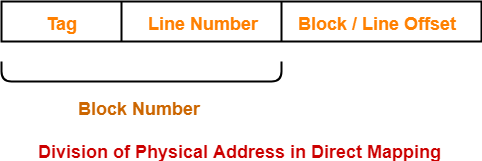## Direct Mapped Cache-

 Direct mapped cache employs direct cache mapping technique.

The following steps explain the working of direct mapped cache-

After CPU generates a memory request,

• The line number field of the address is used to access the particular line of the cache.
• The tag field of the CPU address is then compared with the tag of the line.
• If the two tags match, a cache hit occurs and the desired word is found in the cache.
• If the two tags do not match, a cache miss occurs.
• In case of a cache miss, the required word has to be brought from the main memory.
• It is then stored in the cache together with the new tag replacing the previous one.

## Implementation-

The following diagram shows the implementation of direct mapped cache-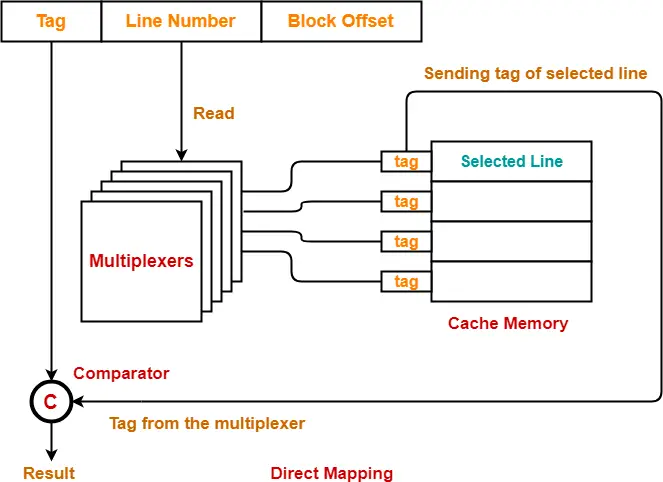(For simplicity, this diagram shows does not show all the lines of multiplexers)

The steps involved are as follows-

## Step-01:

• Each multiplexer reads the line number from the generated physical address using its select lines in parallel.
• To read the line number of L bits, number of select lines each multiplexer must have = L.

## Step-02:

• After reading the line number, each multiplexer goes to the corresponding line in the cache memory using its input lines in parallel.
• Number of input lines each multiplexer must have = Number of lines in the cache memory

## Step-03:

• Each multiplexer outputs the tag bit it has selected from that line to the comparator using its output line.
• Number of output line in each multiplexer = 1.

## UNDERSTAND

It is important to understand-

• A multiplexer can output only a single bit on output line.
• So, to output the complete tag to the comparator,

Number of multiplexers required = Number of bits in the tag

• Each multiplexer is configured to read the tag bit at specific location.

## Example-

• 1st multiplexer is configured to output the first bit of the tag.
• 2nd multiplexer is configured to output the second bit of the tag.
• 3rd multiplexer is configured to output the third bit of the tag and so on.

So,

• Each multiplexer selects the tag bit of the selected line for which it has been configured and outputs on the output line.
• The complete tag as a whole is sent to the comparator for comparison in parallel.

## Step-04:

• Comparator compares the tag coming from the multiplexers with the tag of the generated address.
• Only one comparator is required for the comparison where-

Size of comparator = Number of bits in the tag

• If the two tags match, a cache hit occurs otherwise a cache miss occurs.

## Hit latency-

The time taken to find out whether the required word is present in the Cache Memory or not is called as hit latency.

For direct mapped cache,

 Hit latency = Multiplexer latency + Comparator latency

## Important Results-

Following are the few important results for direct mapped cache-

• Block j of main memory can map to line number (j mod number of lines in cache) only of the cache.
• Number of multiplexers required = Number of bits in the tag
• Size of each multiplexer = Number of lines in cache x 1
• Number of comparators required = 1
• Size of comparator = Number of bits in the tag
• Hit latency = Multiplexer latency + Comparator latency

To gain better understanding about direct mapping,

Watch this Video Lecture

Next Article- Practice Problems On Direct Mapping

Get more notes and other study material of Computer Organization and Architecture.

Watch video lectures by visiting our YouTube channel LearnVidFun.

## Cache Memory-

Before you go through this article, make sure that you have gone through the previous article on Cache Memory.

We have discussed-

 Cache memory bridges the speed mismatch between the processor and the main memory.

When cache hit occurs,

• The required word is present in the cache memory.
• The required word is delivered to the CPU from the cache memory.

When cache miss occurs,

• The required word is not present in the cache memory.
• The page containing the required word has to be mapped from the main memory.
• This mapping is performed using cache mapping techniques.

## Cache Mapping-

• Cache mapping defines how a block from the main memory is mapped to the cache memory in case of a cache miss.

OR

• Cache mapping is a technique by which the contents of main memory are brought into the cache memory.

The following diagram illustrates the mapping process-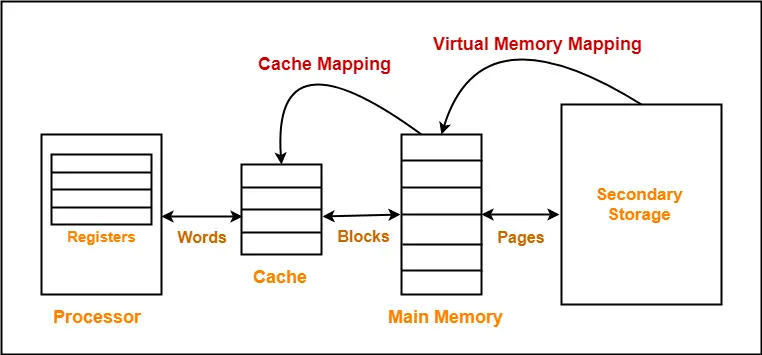Now, before proceeding further, it is important to note the following points-

## NOTES

• Main memory is divided into equal size partitions called as blocks or frames.
• Cache memory is divided into partitions having same size as that of blocks called as lines.
• During cache mapping, block of main memory is simply copied to the cache and the block is not actually brought from the main memory.

## Cache Mapping Techniques-

Cache mapping is performed using following three different techniques-1. Direct Mapping
2. Fully Associative Mapping
3. K-way Set Associative Mapping

## 1. Direct Mapping-

In direct mapping,

• A particular block of main memory can map only to a particular line of the cache.
• The line number of cache to which a particular block can map is given by-

 Cache line number = ( Main Memory Block Address ) Modulo (Number of lines in Cache)

## Example-

• Consider cache memory is divided into ‘n’ number of lines.
• Then, block ‘j’ of main memory can map to line number (j mod n) only of the cache.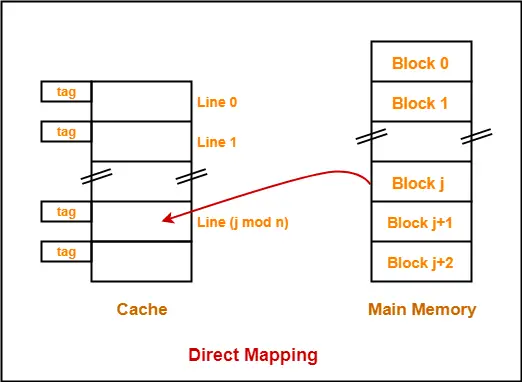## Need of Replacement Algorithm-

In direct mapping,

• There is no need of any replacement algorithm.
• This is because a main memory block can map only to a particular line of the cache.
• Thus, the new incoming block will always replace the existing block (if any) in that particular line.

In direct mapping, the physical address is divided as-## 2. Fully Associative Mapping-

In fully associative mapping,

• A block of main memory can map to any line of the cache that is freely available at that moment.
• This makes fully associative mapping more flexible than direct mapping.

## Example-

Consider the following scenario-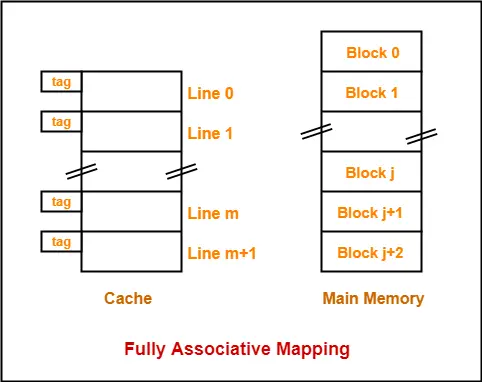Here,

• All the lines of cache are freely available.
• Thus, any block of main memory can map to any line of the cache.
• Had all the cache lines been occupied, then one of the existing blocks will have to be replaced.

## Need of Replacement Algorithm-

In fully associative mapping,

• A replacement algorithm is required.
• Replacement algorithm suggests the block to be replaced if all the cache lines are occupied.
• Thus, replacement algorithm like FCFS Algorithm, LRU Algorithm etc is employed.

In fully associative mapping, the physical address is divided as-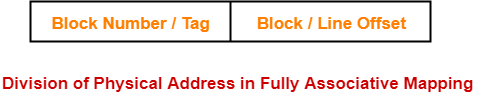## 3. K-way Set Associative Mapping-

In k-way set associative mapping,

• Cache lines are grouped into sets where each set contains k number of lines.
• A particular block of main memory can map to only one particular set of the cache.
• However, within that set, the memory block can map any cache line that is freely available.
• The set of the cache to which a particular block of the main memory can map is given by-

 Cache set number = ( Main Memory Block Address ) Modulo (Number of sets in Cache)

## Example-

Consider the following example of 2-way set associative mapping-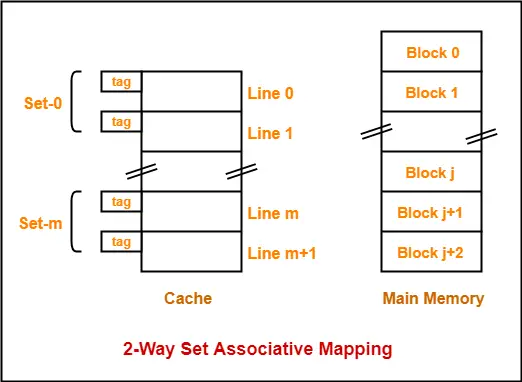Here,

• k = 2 suggests that each set contains two cache lines.
• Since cache contains 6 lines, so number of sets in the cache = 6 / 2 = 3 sets.
• Block ‘j’ of main memory can map to set number (j mod 3) only of the cache.
• Within that set, block ‘j’ can map to any cache line that is freely available at that moment.
• If all the cache lines are occupied, then one of the existing blocks will have to be replaced.

## Need of Replacement Algorithm-

• Set associative mapping is a combination of direct mapping and fully associative mapping.
• It uses fully associative mapping within each set.
• Thus, set associative mapping requires a replacement algorithm.

In set associative mapping, the physical address is divided as-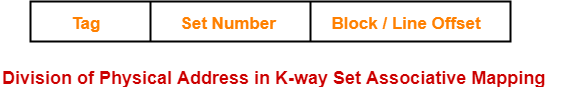## Special Cases-

• If k = 1, then k-way set associative mapping becomes direct mapping i.e.

 1-way Set Associative Mapping ≡ Direct Mapping

• If k = Total number of lines in the cache, then k-way set associative mapping becomes fully associative mapping.

Next Article- Direct Mapping | Implementation & Formulas

Get more notes and other study material of Computer Organization and Architecture.

Watch video lectures by visiting our YouTube channel LearnVidFun.